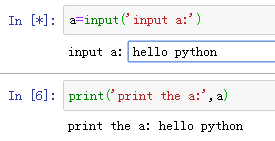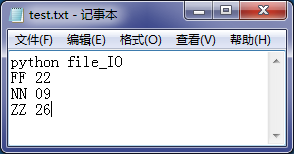【PYTHON】语法基础 | 开始使用Python – Python量化投资

# 【PYTHON】语法基础 | 开始使用Python

Python的热度不言而喻，机器学习、数据分析的首选语言都是Python，想要学习Python的小伙伴也很多，我之前也没有认真用过Python，所以也想体验一下它的魅力，索性花了两天集中看了一下它的基本语法，组织了这篇笔记，一是加强一下自己的记忆和理解，二是可以分享给共同学习的小伙伴。这篇笔记主要是Python的基本语法，算是入个门，后面可以边用边加强。

## 输入与输出• Input()接收的输入数据都认为是字符串，如做其它类型使用，需转换。

• Print()可以做格式化输出，同C类似，%d（整型）%s（字符串）%f（浮点型），如搞不清，直接都用%s也可以，它会把任何数据类型转换为字符串。

```#用python计算圆的面积；
#定义一个变量接收输入的圆半径
r=input("请输入圆半径：")
#定义一个变量存放计算出的圆面积
#特别注意：python3针对输入统一都认为是字符串型
#所以在计算时要将其转换为整形数，格式：int（x）
s=3.14*int(r)*int(r)
#输出计算出的圆面积s
print("圆面积s=",s)
#也可以直接在输出print里计算圆面积
print("直接在print里计算面积：",3.14*int(r)*int(r))
#利用占位符实现格式化输入，这里和C语言是类似的
print("输入的圆半径是%s,圆面积是%f" %(r,3.14*int(r)*int(r)))```

```运行结果：

## 变量与数据类型

```#include<iostream>
#include<string>
#include <limits>
using namespace std;

int main()
{
int a=10;  //整型：4个字节
char b='x';  //字符型：1个字节
float c=3.14; //单精度浮点：4个字节，7个数字

cout<<"int a="<<a<<"\t长度是"<<sizeof(int)<<endl
<<"char b="<<b<<"\t长度是"<<sizeof(char)<<endl
<<"float c="<<c<<"\t长度是"<<sizeof(float)<<endl;
}```

```运行结果：
int a=10       长度是4
char b=x       长度是1
float c=3.14   长度是4```

```#定义一个变量a，给它一个整形值27
a=27
print(a)
#再将a的值变为一个浮点数，3.1415927
a=3.1415927
print(a)
#再将a的值变为一个字符串，J_ZHANG
a="J_ZHANG"
print(a)```

```运行结果：
27
3.1415927
J_ZHANG```

```a = 123
#执行这句话，python干了两件事
#一是在内存中建了个123的整数
#二是在内存中建了个变量a，然后将a指向刚建的整数123
b = a
#执行这句话，python建了个变量b，并指向a所指向的123
a = 'ZNN'
#这句话python建了个字符串ZNN，并将a指向了新建的ZMM
#注意：b没有改变哟，它还是指向a原来指向的那个123
print("执行输出：a=",a,"；b=",b)
#print(b)```

```运行结果：

🔗动态语言：像Python这样，变量使用之前不需要类型声明，变量的类型就是被赋值的那个值的类型，动态语言是在运行时确定数据类型的语言。

🔗静态语言：在定义变量时必须指定变量类型，且在赋值时类型要相匹配，否则会出现运行时类型错误。如C/C++/JAVA等语言。

## List（列表）

```#定义一个list变量stuName，list元素可为不同类型
stuName=['FF','NN','ZZ',1999,'HH','XY']
#获取list的长度，len()
print('stuName的元素个数：',len(stuName))
print(stuName)
#访问list元素，索引是从0开始，反向访问从-1开始，-1是最后一个元素索引
print('第一个元素是:%s，倒数第二个元素是:%s' %(stuName,stuName[-2]))
#增、删、改
stuName.append('J_ZHANG')#在末尾追加一个元素
print(stuName)
stuName.insert(0,2009)#在指定位置插入元素
print(stuName)
stuName.pop()#删除最后一个元素
print(stuName)
stuName.pop(4)#删除第五个元素
print(stuName)
stuName='J_ZHANG'#修改索引为3的元素
print(stuName)
stuInfo=[stuName,[10,20,30]]#嵌套使用
print(stuInfo)
print(stuInfo)#二维List的访问方式```

```运行结果：
stuName的元素个数：6
['FF', 'NN', 'ZZ', 1999, 'HH', 'XY']

['FF', 'NN', 'ZZ', 1999, 'HH', 'XY', 'J_ZHANG']
[2009, 'FF', 'NN', 'ZZ', 1999, 'HH', 'XY', 'J_ZHANG']
[2009, 'FF', 'NN', 'ZZ', 1999, 'HH', 'XY']
[2009, 'FF', 'NN', 'ZZ', 'HH', 'XY']
[2009, 'FF', 'NN', 'J_ZHANG', 'HH', 'XY']
[[2009, 'FF', 'NN', 'J_ZHANG', 'HH', 'XY'], [10, 20, 30]]
J_ZHANG```

## Tuple（元组）

```#定义一个Tuple变量stuName，元素可为不同类型，但值不可改变
stuName=('FF','NN','ZZ',1999,'HH','XY')
print(stuName)
print(stuName,stuName[-1])#Tuple元素访问与List类似
#stuName='J_ZHANG' #会报错，值不可变
stuInfo=('aa','bb',[10,20,'string'])#Tuple里面包含List类型元素
print(stuInfo)
stuInfo=30#包含的List里的元素是可以修改的
print(stuInfo)```

```运行结果：
('FF', 'NN', 'ZZ', 1999, 'HH', 'XY')
FF XY
('aa', 'bb', [10, 20, 'string'])
('aa', 'bb', [10, 20, 30])```

## Dictionary（字典）

```#定义一个Dict变量stuScore，姓名（key）与成绩（value）一一对应
stuScore={'FF':80,'NN':90,'ZZ':100}
stuScore['HH']=70#在后面添加一个键值对
print(stuScore)
print(stuScore['HH'])#获取键所对应的值
stuScore.pop('HH')#删除指定的键值对
stuScore['ZZ']=89#修改键ZZ的值
print(stuScore)
print(stuScore.keys())#获取所有键
print(stuScore.values())#获取所有值
print(stuScore.items())#按组打印```

```运行结果：
{'FF': 80, 'NN': 90, 'ZZ': 100, 'HH': 70}
70
{'FF': 80, 'NN': 90, 'ZZ': 89}
dict_keys(['FF', 'NN', 'ZZ'])
dict_values([80, 90, 89])
dict_items([('FF', 80), ('NN', 90), ('ZZ', 89)])```

```#定义一个Dict变量stuInfo，姓名（key）与性别（value）
stuInfo={'FF':'女','NN':'女','ZZ':'男'}
#下面统计 stuInfo 里面'男'、'女'的数量
stuXBTJ={}#定义一个空字典用于存放统计结果
for xb in stuInfo.values():#遍历
if xb in stuXBTJ:#如果该性别已存在，则加1
stuXBTJ[xb]= stuXBTJ[xb] + 1
else:#否则，该性别数量初始化为1
stuXBTJ[xb]=1
print(stuXBTJ)#打印结果```

```运行结果：
{'女': 2, '男': 1}```

## Set（集合）

```#利用一个List创建一个Set集合
stu=['FF','ZZ','NN','JJ','ZZ','FF']
s=set(stu)#创建集合s
print(s)#打印s发现重复项已被自动过滤
s.add(27)#添加一项
s.remove('JJ')#删除一项
print(s)
s1=set([1,2,3,4,5])
s2=set([4,5,6,7,8])
print('交集',s1 & s2)#计算两个集合的交集
print('并集',s1 | s2)#计算两个集合的并集
print('差集',s1 - s2)#计算两个集合的差集```

```运行结果：
{'NN', 'ZZ', 'JJ', 'FF'}
{'NN', 'ZZ', 27, 'FF'}

## 选择与循环

```score=78
if score>=60:
print('及格！')#执行
print('OK ！')#执行，没有大括号，用缩进
else:
print('不及格！')

if score>=80:
print('优秀！')
elif score>=70:
print('良好！')#执行
elif score>=60:
print('及格！')
else:
print('差！')
#if...elif是从上到下判断，遇到true即执行，其余忽略。```

```运行结果：

OK ！

```#for循环实现list遍历
stuName=('FF','NN','ZZ')
for name in stuName:
print(name)

stuInfo=[['FF','NN'],[22,27]]
for i in stuInfo:#Ffor循环嵌套使用
for j in i:
print(j)

sum=0
n=0
while n<=10:#while循环的使用
sum=sum+n
n=n+1
print(sum)```

```运行结果：
FF
NN
ZZ
FF
NN
22
27
55```

break和continue的用法与C++一致。

break：提前结束循环。

continue：结束本轮循环，直接开始下一轮循环。

## 文件读写

• 文件打开用完要关闭，open()和close()配对使用。

• 避免文件打开失败或忘记关闭等造成的错误，使用with…as…操作文件。```#使用open()函数读文件，文件位置：D:\test.txt
f=open("D:\\test.txt","r")#打开文件，第二个参数“r”表示读
fn=f.read()
print(fn)
f.close()#完成操作后必须要关闭文件

#文件打开用完就要关闭，但不排除一通操作后忘了关闭文件，
#同时还存在文件打开失败的情况，使用with…as…操作文件更可靠，更简洁。
with open("D:\\test.txt","r") as f:
fn=f.read()
print(fn)```

```运行结果：
python file_IO
FF 22
NN 09
ZZ 26
python file_IO
FF 22
NN 09
ZZ 26```

• 写文件时，如果目标位置没有文件，python会创建文件。

• 如果目标位置有文件，参数“w”就会覆盖写入，追加写入可用参数“a”。

• 可以反复调用write()写数据，但如果最后忘记close()文件，后果可能是数据丢失，保险起见，还是使用with…as…更靠谱。

```#在D盘创建一个新文件test_new.txt,然后写入内容，
f=open("D:\\test_new.txt","w")
f.write("J_ZHANG")
f.close()
#再次写入就会覆盖原内容
with open("D:\\test_new.txt","w") as f:
f.write("覆盖了吗？")
f.write("\n")#换一下行
#使用参数“a”实现追加写入
with open("D:\\test_new.txt","a") as f:
f.write("J_ZHANG追加写入的。")
#打印出来看一下
with open("D:\\test_new.txt","r") as f:
print(f.read())```

```运行结果：

J_ZHANG追加写入的。```

## 定义与使用函数

Python用def关键字定义一个函数，定义时需确定函数名与参数，函数可以有多个返回值，但如函数没有return，则默认还回none。因python变量为动态类型，如函数对参数有类型要求，需在函数体内进行类型检查。

```#使用def定义一个函数：求圆面积
def cir_area(r):
if not isinstance(r,(int,float)):#参数类型检查，r为整型或浮点型
raise TypeError("参数r类型错误！")
if r<=0:
return 0#判断如果半径小于等于0则返回0
else:
return 3.14*r*r

#调用函数
s=cir_area(4)#半径为4的圆面积
print(s) ```

```运行结果：
50.24```

OK，就酱紫！暂时先到这儿。

————————– END ————————–

https://www.cnblogs.com/nnzhang/p/11341495.html

「点点赞赏，手留余香」

还没有人赞赏，快来当第一个赞赏的人吧！
TensorFlow

0 条回复 A 作者 M 管理员
所有的伟大，都源于一个勇敢的开始！